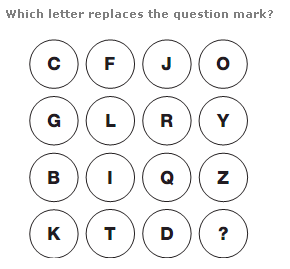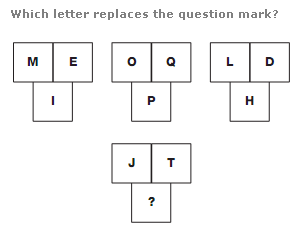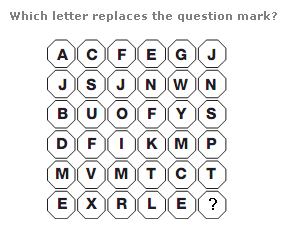# Puzzles - Missing letters puzzles

### Exercise :: Missing letters puzzlesAnswer : O Explanation : Working in rows, from left to right, in the top row add 3 to the numerical value of the first letter to give the next one along, then 4, then 5. In the next row, add 5 to the first letter, then 6, then 7. In the third row, start by adding 7, then 8, then 9, and in the bottom row, add 9, then 10, then 11.Answer : O Explanation : In each group of 3 boxes, the numerical value in the lower box equals the average of the numerical values of the letters in the top 2 boxes.Answer : Y Explanation : Split the diagram into equal quarters of 3x3 squares. In each quarter, move around the spaces in a clockwise spiral towards the centre, starting on the top left. Letters follow the alphabetic sequence, In steps of 2 letters, then 3, then 4 etc.